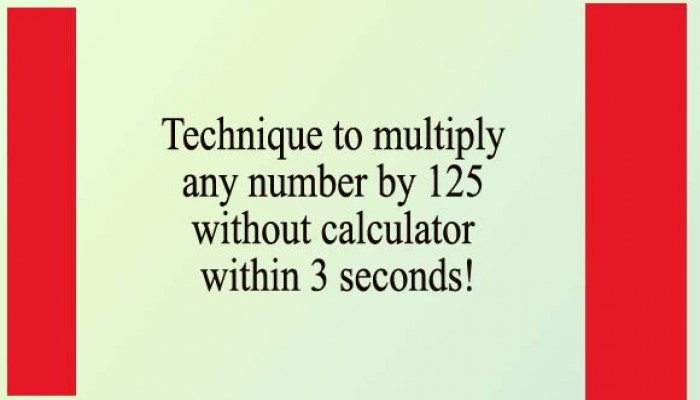Are you prepared well enough for job examination, admission test or any competitive examinations?

You know in this kind of examination, using calculator is prohibited. But you will have not enough time to solve the numerical problems.

Here is tip to find out the multiplication result of any number by 125. Look at the example.

Example 01: Multiply 72 by 125 within 3 seconds

Technique: Divide 1000 by 125, it is 8, right? (1000/125=8)

Always, divide the number (except 125) by 8, then put "000" (3 zeros) after the result.

Solution:

Divide 72 by 8, it is 9

Now, write 9 first, and put "000" (3 Zeros) after 9,

That means, 72x125=9000

Example 02: Multiply 112 by 125 within 3 seconds

112x125=?

Divide 112 by 8, it is 14,

Now write 14 first, and put "000" (3 Zeros) after 14,

That means, 112x125=14000

Example 03: Multiply 56 by 125 within 3 seconds

Divide 56 by 8, it is 7, now write 7 first, and put "000" (3 Zeros) after 7,

That means, 56x125=7000

Now start practicing. Off course, within 3 seconds you can find out the result.